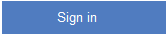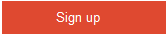# Explain where you can use functions and variables?

0 votes
8 viewsposted Mar 11
Share this question## 1 Answer

0 votes

A function tries to define these relationsips. It tries to give the relationship a mathematical form. An equation is a mathematical way of looking at the relationship between concepts or items. These concepts or items ar represented by what are called variables.
A variable represents a concept or an item whose magnitude can be represented by a number, i.e. measured quantitatively. Variables are called variables because they vary, i.e. they can have a variety of values. Thus a variable can be considered as a quantity which assumes a variety of values in a particular problem. Many items in economics can take on different values. Mathematics usually uses letters from the end of the alphabet to represent variables. Economics however often uses the first letter of the item which varies to represent variables. Thus p is used for the variable price and q is used for the variable quantity.answer Mar 12
Similar Questions